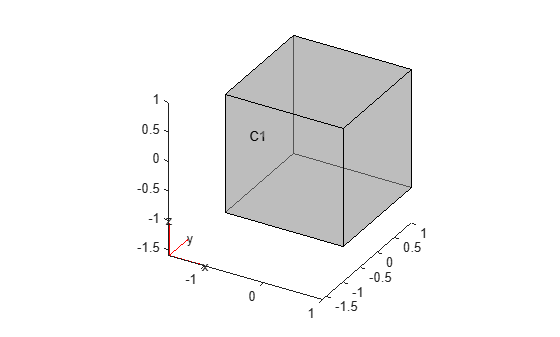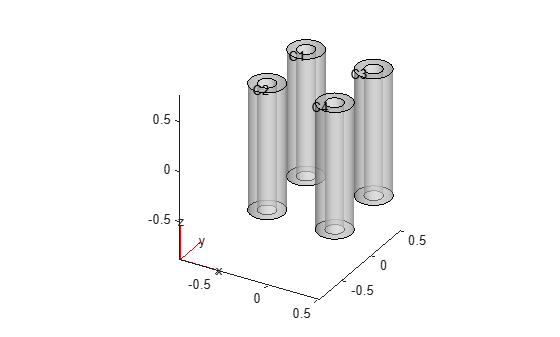Create void regions inside 3-D geometry

## Syntax

``g3 = addVoid(g1,g2)``

## Description

example

````g3 = addVoid(g1,g2)` creates void regions inside `g1` using all cells of `g2`. All cells of the geometry `g2` must be contained inside one cell of the geometry `g1`. Ensure that the geometries do not have enclosed cavities and do not intersect one another. NoteAdded void regions modify a geometry, but they do not modify the corresponding mesh. After modifying a geometry, always call `generateMesh` to ensure a proper mesh association with the new geometry. ```

## Examples

collapse all

Create and plot a geometry.

```g1 = multicuboid(2,2,2,'Zoffset',-1); pdegplot(g1,'CellLabels','on','FaceAlpha',0.5)```Import and plot another geometry.

```g2 = importGeometry('DampingMounts.stl'); pdegplot(g2,'CellLabels','on','FaceAlpha',0.5)```Scale and move the second geometry to fit entirely within the cube `g1`.

```g2 = scale(g2,[1/1500 1/1500 1/100]); g2 = translate(g2,[-0.5 -0.5 -0.5]);```

Plot the result.

`pdegplot(g2,'CellLabels','on','FaceAlpha',0.5)`Create void regions inside the cube using the cells of the geometry `g2`. Plot the result.

```g3 = addVoid(g1,g2); pdegplot(g3,'CellLabels','on','FaceAlpha',0.4)```## Input Arguments

collapse all

3-D geometry, specified as a `DiscreteGeometry` object. For more information, see DiscreteGeometry Properties.

3-D geometry, specified as a `DiscreteGeometry` object. For more information, see DiscreteGeometry Properties.

## Output Arguments

collapse all

Resulting 3-D geometry, returned as a `DiscreteGeometry` object. For more information, see DiscreteGeometry Properties.